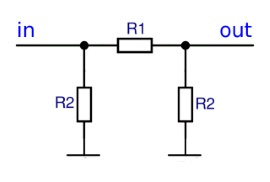# Pi Attenuator

Online calculator and formulas for calculating the resistances of a Pi attenuator

## Calculate the Pi Attenuator

In the case of attenuators for high frequencies, attention must be paid to the impedance matching. The input impedance and the output impedance must be equal to the wave impedance of the lines.

On this side, a pi attenuator is calculated whose resistances, i.e. also the impedance, are the same on the input and output sides.

If the resistances on the input and output sides are not the same, an impedance matching takes place at the same time as the attenuation.

Pi Attenuator calculator

 Should voltage ratio or damping be entered ? Attenuation in db Voltage ratio U1 / U2 Input Impedance Attenuation in db Voltage U1 Voltage U2 Decimal places 0 1 2 3 4 6 8 10 Result Resistance R1: Resistance R2:## Formulas for the Pi Attenuator

The resistances R1 and R2 of the PI attenuator are calculated from the impedance Z and the attenuation factor a. The damping factor a is calculated from the ratio of the output voltage to the input voltage (U1 / U2), or the attenuation measure ΔL in db.

$$\displaystyle a=\frac{U_1}{U_2}= 10^{\frac{ΔL}{20 db}}$$
$$\displaystyle R_1= Z\frac{a^2-1}{2a}$$
$$\displaystyle R_2= Z\frac{a+1}{a-1}$$